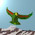## Thursday, October 27, 2011

### Theory Update 120

Now we should start applying quark lepton complementarity to the MNS and CKM mixing matrices, starting with the $\theta_{13} = 0$ tribimaximal form. Today's observation is that, using the $R_2$ factor parameters

CKM: $(-0.231,24,0.0035)$
MNS: $(1,\sqrt{2},0)$

the resulting matrices have very similar eigenvalues. That is,

CKM: $-0.9651 - 0.2621 i$, $0.4813 \pm 0.8765 i$
MNS: $-0.9856 + 0.1691 i$, $0.4928 \pm 0.8701 i$

where the first eigenvalue is the determinant, and the real and imaginary parts of the determinant for the MNS matrix are exactly $(\pm \sqrt{2} - 1)/ \sqrt{6}$.

Using the known CKM parameters, and matching the determinants, we could then determine a small $\theta_{13}$ for the neutrino mixing matrix.

#### 4 comments:

1.So now surely those of you with a decent algebra package can fix up the parameter sets quick smart, no?

2.No, a large $c = -10.5$ works better, in flipping the determinant by a phase $\pi/2$. That would give a $\theta_{13}$ of around $0.095$, which is in the right range.

3.That is, the value that will be accurately measured in the next couple of years may be around $\sin^{2} 2 \theta_{13} = 0.036$.

4.Oh, look. That value sits at the centre of the MINOS range.

Note: Only a member of this blog may post a comment.